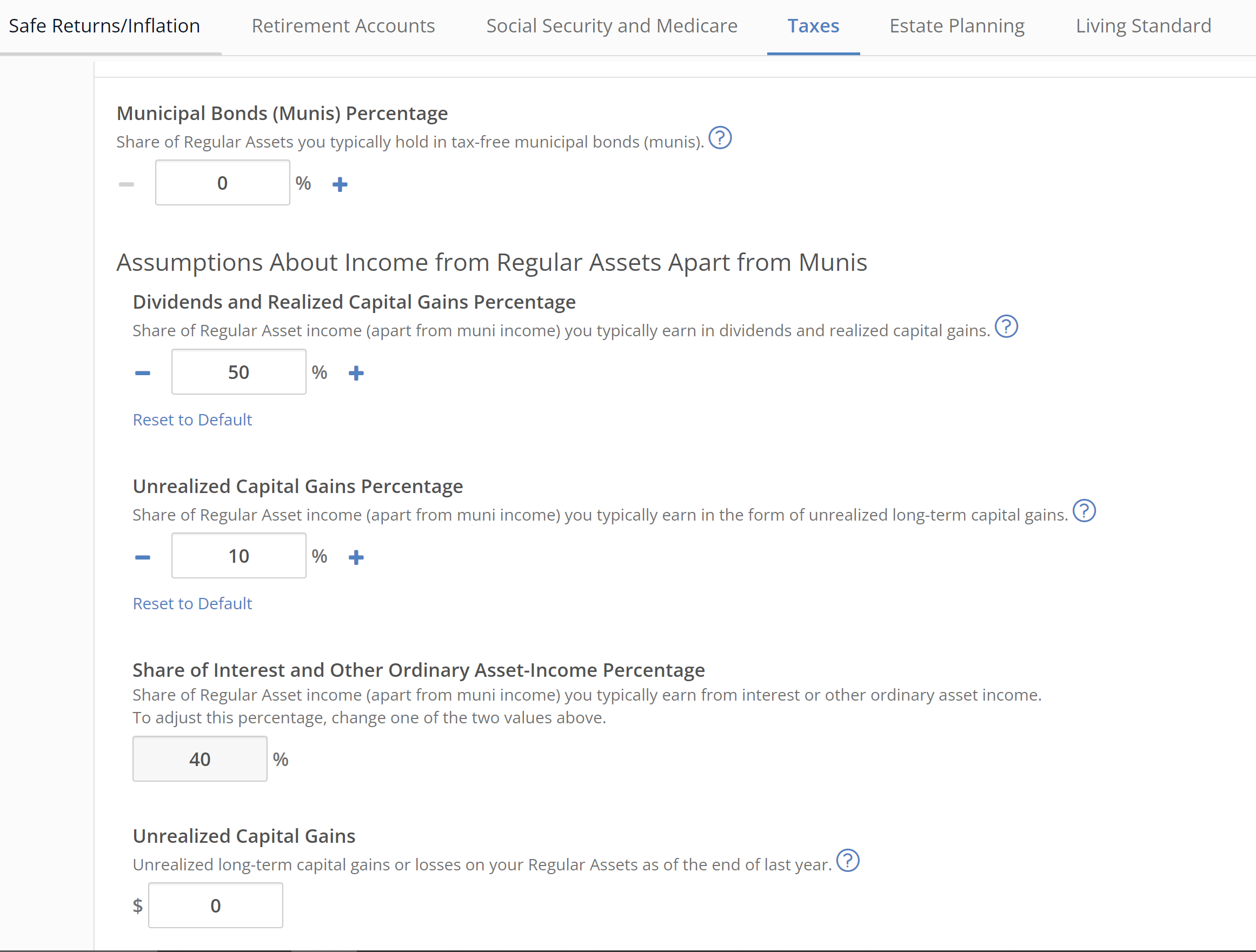Regular assets (non-retirement assets) might generate ordinary interest or qualified dividends and capital gains.

You can enter the typical percentage of current-year and all future year's annual total return that you want to be treated as qualified dividends/capital gains in Settings > Taxes  > Dividends and Realized Capital Gains Percentage. If your regular assets generated \$80 in total return and 50% of that was from distributed capital gains or qualified dividends, you should enter 50%.

This help page below focuses on the income generated when an investor sells a security and thus realizes some portion of the accrued unrealized gains.

For help with other fields related to these settings, see here: Dividends and Distributions Percentage

MaxiFi views withdrawals from regular assets as an opportunity to generate a capital gain. These withdraws will trigger capital gains tax and MaxiFi will realize these capital gains along with any yet unrealized capital gains on a pro-rata basis when they are withdrawn from the regular asset account. Your Annual Saving and Withdrawal Report shows the pattern of annual saving and withdrawal over the life of your model.

Such capital gains are realized on a pro-rata basis when they are withdrawn from the regular asset account and we exempt death bequests from capital gains.

You set unrealized capital gains (i.e. previously accumulated long-term capital gains or losses) on your Regular Assets as of the end of last year through Settings and Assumptions > Taxes tab. Unrealized long-term capital gains are positive dollar amounts and carryover losses are negative dollar amounts.In the image above, the user is indicating that 50% of future earnings on regular assets (i.e., the annual percentage of total return on regular assets) will be taxable annually at the capital gains and qualified dividends rate. And 10% of total return will be capital appreciation and accrue and then taxed when realized as its realized (withdrawn). The remaining 40% of total return will thus be taxable annually as ordinary income. The user is also indicating that \$0 of the existing current balance in regular assets represents unrealized capital gains.

With this information, MaxiFi each year going forwards updates both unrealized capital gains (carryover losses if negative) and the Regular Asset balance at the end of the year based on the annual return. Positive returns that include capital gains increase unrealized capital gains or decrease carryover losses. Negative returns decrease unrealized capital gains or increase carryover losses. When MaxiFi generates a withdrawal from the Regular Asset account and there are unrealized gains, then the pro-rata share of capital gains realized in that withdrawal is determined based on the updated end of year balances and the amount of the withdrawal. Carryover losses are used to offset capital gains and to take up to a \$3,000 loss per year. MaxiFi now passes bequests to heirs with no reduction due to capital gains taxation. That is, it now incorporates what's known as "step up in basis."

(The capital gains on real estate property are realized when you sell the property.)

There are four possible permutations as illustrated in the following examples.

In all cases, last year's (2018 in this example) Regular Asset Balance is \$300,000, the percent of taxable Regular Assets income that are long term capital gains or qualified dividends is 80%, and the current-year withdrawal (2019 in this example) is \$50,000.

1. 2018 Unrealized capital gains: \$200,000 and 2019 Nominal Return: 3.5%.

• First, Balances based only on 2019 returns:
• Regular assets: \$310,500 = \$300,000 + 3.5% *\$300,000.
• Unrealized capital gains: \$208,400 = \$200,000 + 3.5% * \$300,000 * 80%.
• 2019 Capital gains:
• Realized Capital Gains: \$33,559 = \$50,000 * (\$208,400 / \$310,500).
• EOY 2019 balances:
• Regular Assets: \$260,500 = \$310,500 - \$50,000.
• Unrealized Capital Gains: \$174,841 = \$208,400 - \$33,559.
2. 2018 Unrealized Capital Gains: \$200,000 and 2019 Nominal Return: - 3.5%.
• First, Balances based only on 2019 returns:
• Regular assets: \$289,500 = \$300,000 - 3.5% *\$300,000.
• Unrealized capital gains: \$191,600 = \$200,000 - 3.5% * \$300,000 * 80%.
• 2019 Capital gains:
• Realized Capital Gains: \$33,092 = \$50,000 * (\$191,600 / \$289,500).
• EOY 2019 balances:
• Regular Assets: \$239,500 = \$289,500 - \$50,000.
• Unrealized Capital Gains: \$158,508 = \$191,600 - \$33,092.
3. 2018 Carryover losses: -\$200,000, i.e. negative gains and 2019 Nominal Return: 3.5%.
• First, Balances based only on 2019 returns:
• Regular assets: \$310,500 = \$300,000 + 3.5% *\$300,000.
• Unrealized capital gains: -\$191,600 = -\$200,000 + 3.5% * \$300,000 * 80%.
• 2019 Capital gains:
• Realized Capital Gains: \$30,853 = \$50,000 *(\$191,600 / \$310,500).
• EOY 2019 balances:
• Regular Assets: \$260,500 = \$310,500 - \$50,000.
• Unrealized Capital Gains: \$160,747 = \$191,600 - \$30,853
4. 2018 Carryover losses: -\$200,000, i.e. negative gains and 2019 Nominal Return: -3.5%.
• First, Balances based only on 2019 returns:
• Regular assets: \$289,500 = \$300,000 - 3.5% *\$300,000.
• Unrealized capital gains: -\$208,400 = -\$200,000 - 3.5% * \$300,000 * 80%.
• 2019 Capital gains:
• Realized Capital Gains: \$35,993 = \$50,000 * (\$208,400 / \$289,500).
• EOY 2019 balances:
• Regular Assets: \$239,500 = \$289,500 - \$50,000.
• Unrealized Capital Gains: \$172,407 = -\$208,500 - \$35,993.